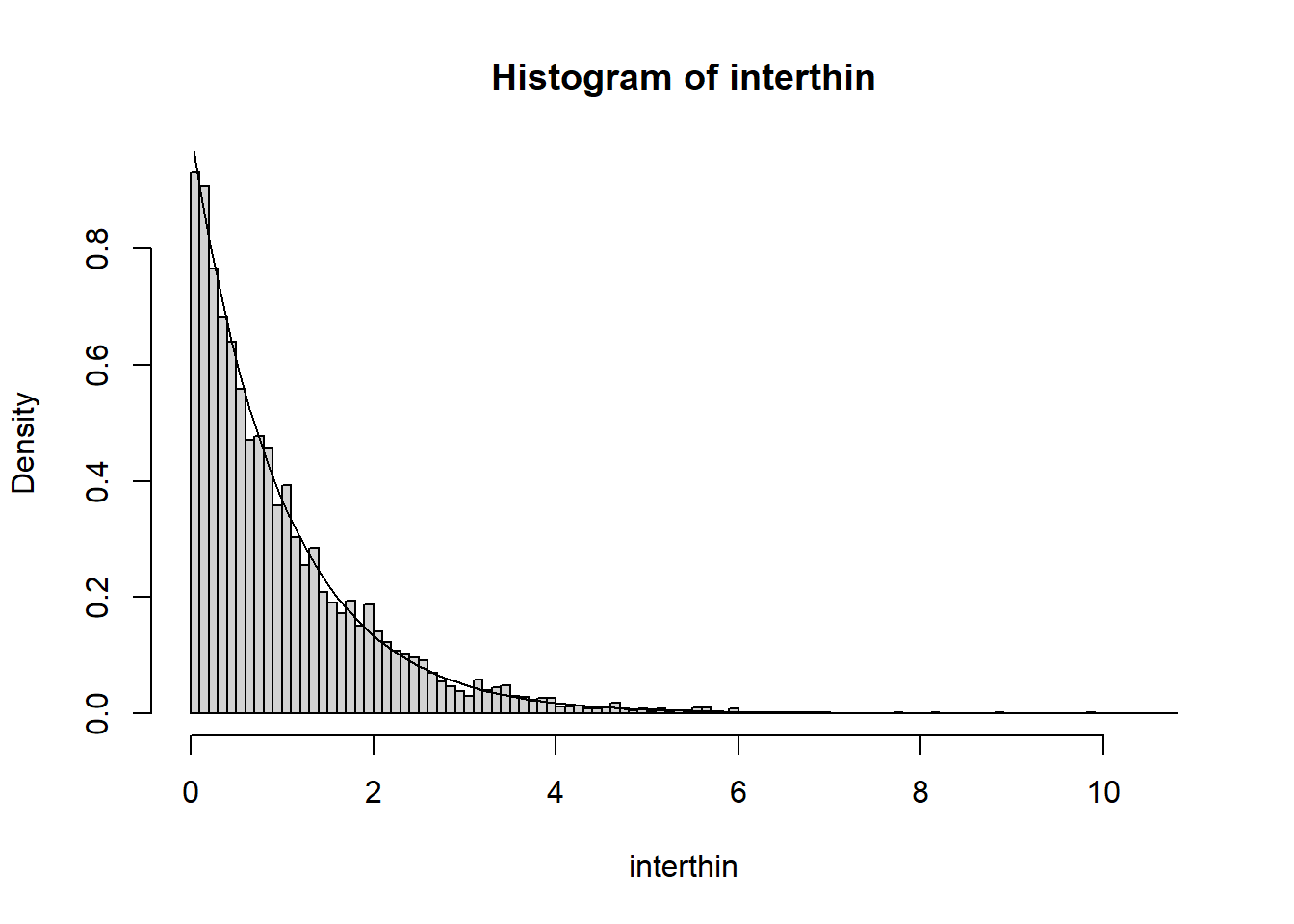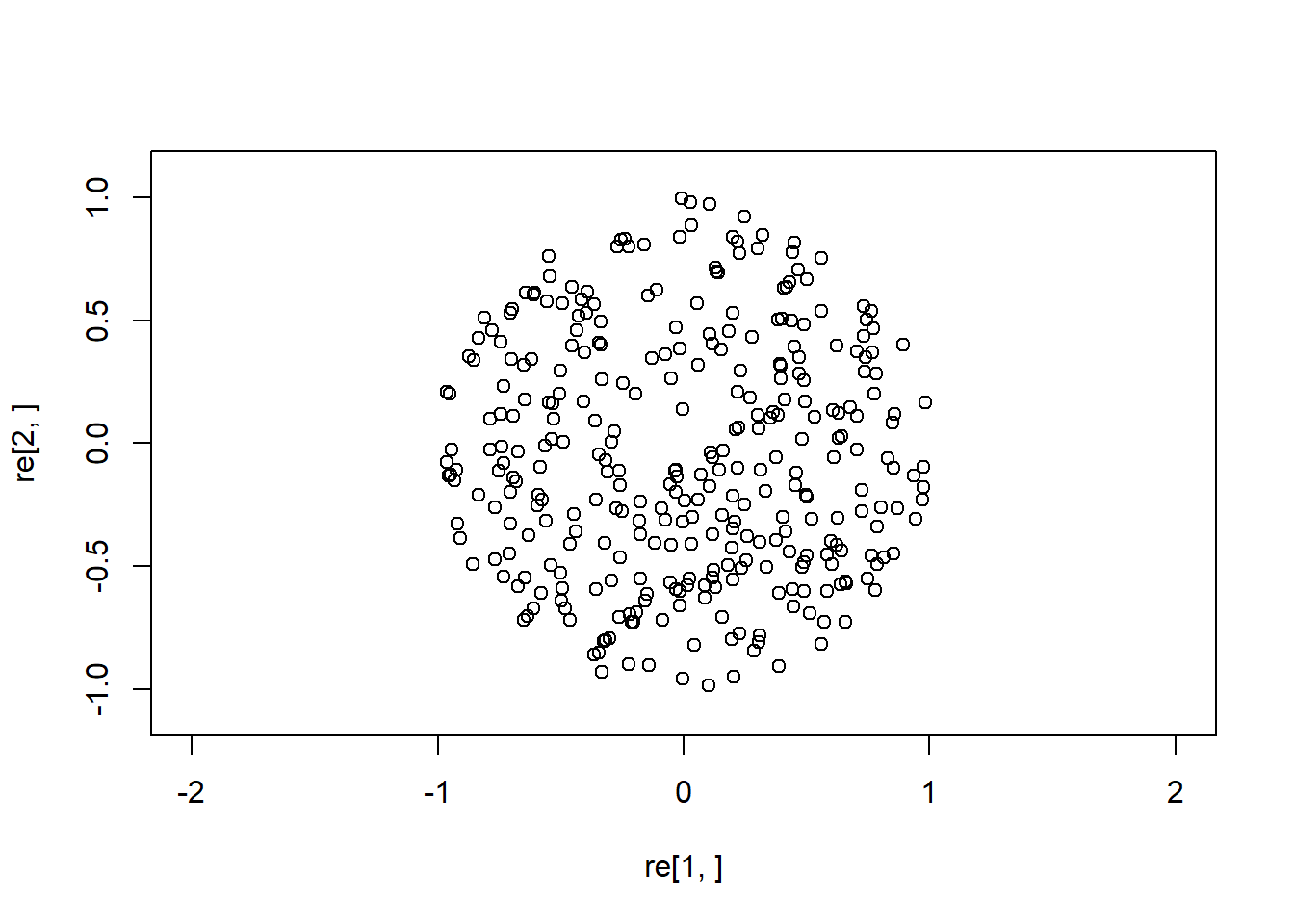Exercise 1 (Deterministic colouring) Let $$\Pi$$ a Poisson point process on $$[0, \infty)$$. Suppose we colour the first arrival blue, and then next arrival red, and continue colouring the points in this alternating fashion. Consider the point processes $$\Gamma$$ formed by considering only the blue points. Is this a Poisson point process? Explain.

Solution. No. The interarrival times between two blue points is given by the sum of two exponential random variables, and is no longer an exponential.

Exercise 2 (Shop keeper) Suppose we model the number of customers that arrive at a high street shop on at particular day by a Poisson process of intensity $$\lambda >0$$, where $$\lambda$$ is measured in customers per hour. We wish to estimate $$\lambda$$. Suppose the shop is really high-end and on some days has no customers, on its $$6$$ hours of operations. The shop keeper only keeps track of whether she had has any customers are not; that is, her records $$x = (x_1, \ldots, x_n)$$ are a binary sequence. Find a consisent estimtor for $$\lambda$$

Solution. Let $$X_i$$ be the binary Bernoulli random variables that corresponds to whether a customer arrived on the $$i$$th day. Note that $$\mathbb{P}(X_i = 0) = e^{-6\lambda}$$ and $$\mathbb{P}(X_i = 1) = 1 - e^{-6\lambda}$$. Assume that these are independent random variables, the law of large numbers gives that $n^{-1}(X_1 + \cdots X_n) \to \mathbb{E}X_1 = 1 - e^{-6\lambda},$ as $$n \to \infty$$. Thus setting $T_n := -\frac{1}{6}\log( 1- n^{-1}(X_1 + \cdots+ X_n)),$ we also know that $$T_n \to \lambda$$, as $$n \to \infty$$, since $$\log$$ is continuous.

Exercise 3 Suppose we $$\Pi$$ is a Poisson point process on $$[0, \infty)$$ of intensity $$\lambda$$. Using the construction of $$\Pi$$ as exponential inter-arrival times, prove that conditioned on the event that the unit interval contains exactly one point, the distribution of the its location is uniform.

Solution. Let $$X_1$$ and $$X_2$$ be independent exponential random variables of rate $$\lambda$$ representing the inter-arrival times. Let $$U$$ be the location of the point in question. We are asked to compute $\mathbb{P}(U \leq x | X_1 < 1, X_2+X_1 >1)=\mathbb{P}(X_1 \leq x | X_1 < 1, X_2+X_1 >1)$ for $$0 < x <1$$.
Since $$X_1$$ and $$X_2$$ are independent, we have $\begin{eqnarray*} \mathbb{P}(X_1 < x, X_2+X_1 >1) &=& \int_0 ^x \lambda e^{- \lambda x_1} \Big( \int_{1-x_1} ^{\infty} \lambda e^{ -\lambda x_2} dx_2 \Big)dx_1 \\ &=&\int_0 ^x \lambda e^{-\lambda x_1} e^{-\lambda(1-x_1)}dx_1 \\ &=& x \lambda e^{-\lambda}. \end{eqnarray*}$ Hence, using this formula in the numerator, and also in the denominator with $$x=1$$, we have $\mathbb{P}(U \leq x | X_1 < 1, X_2+X_1 >1) = \frac{x \lambda e^{-\lambda}}{ \lambda e^{-\lambda}} =x$ as desired.
Exercise 4 (Random deletion) Simulate a Poisson process of intensity $$\lambda=2$$, say with $$10000$$ arrivals. Delete each arrival independently with probability $$p=\tfrac{1}{2}$$ to from a new thinned process. Plot a histogram of the inter-arrival times of the thinned process. What should you see? Why?
Solution. The histogram approximates the exponential density with rate $$1$$, since we know that the resulting thinned process is still a Poisson process with intensity $$p \lambda=1$$.
inter=rexp(10000, 2)
arr = cumsum(inter)
coin = rbinom(length(arr), 1, 0.5)
for (i in 1:length(arr)) if (coin[i]==0){arr[i]<- 0}
arr<-setdiff(arr, 0)
interthin = arr
for (i in 1: length(arr)-1){interthin <- c(interthin, arr[i+1] - arr[i]) }
x = seq(0, max(interthin)+1, by=0.1)
hist(interthin, prob=T, x)
curve(dexp(x), add=T)Exercise 5 (Poisson on a disc) Simulate a Poisson point process of intensity $$100$$ on a disc.

Solution. We simulate a single point on the disc, by simulating a uniform point on a square, and keep it if lands inside an inscribed disc, or repeat otherwise. Then we implent the characterization of Poisson point processes as a Poisson number of uniform points. Note a disc has area $$\pi r^2$$.

point <- function(){
z=2
while(length(z)==1){
x = 2*runif(1) -1
y = 2*runif(1) -1
if (x^2 + y^2 <1) { z<-c(x,y)}
}
z
}
re=replicate(rpois(1,100*pi), point())
plot(re[1,], re[2,], xlim=c(-1.1,1.1), ylim=c(-1.1,1.1), asp=1)